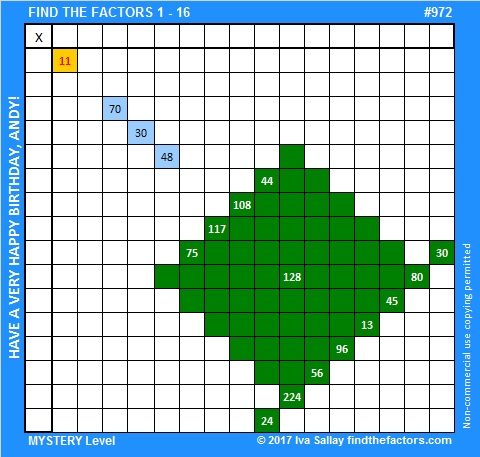# 972 Happy Birthday, Andy!

Today is my brother Andy’s birthday. I know Andy can solve these puzzles so I’ve made him a puzzle cake with factors from 1 to 16. Adding extra factor possibilities complicates the puzzle and makes it a little more difficult to read as a multiplication table, but it is still solvable. Since these puzzles have only one solution and are solved by logic and not by guessing and checking, I added a clue right in the center of the cake to ensure a unique solution. Happy birthday, Andy!Print the puzzles or type the solution in this excel file: 10-factors-968-977

Now I’ll share a little about the number 972 which is the 13th Achilles number.  All of the exponents in its prime factorization are greater than 1, yet the greatest common factor of those exponents is still 1. The previous Achilles number, 968, and 972 are the closest two Achilles numbers so far.

I think 972 has some interesting representations when written in some other bases:

It’s 33030 in BASE 4 because 3(4⁴) + 2(4³) + 0(4²) + 3(4) + 0(1) = 3(256 + 64 + 4) = 3(324) = 972
363 in BASE 17 because 3(17²) + 6(17) + 3(1) = 972
300 in BASE 18 because 3(18²) = 3(324) = 972
RR in BASE 35 (R is 27 base 10) because 27(35) + 27(1) = 27(36) = 972
R0 in BASE 36 because 27(36) + 0(1) = 27(36) = 972

• 972 is a composite number.
• Prime factorization: 972 = 2 × 2 × 3 × 3 × 3 × 3 × 3, which can be written 972 = 2²× 3⁵
• The exponents in the prime factorization are 2 and 5. Adding one to each and multiplying we get (2 + 1)(5 + 1) = 3 × 6 = 18. Therefore 972 has exactly 18 factors.
• Factors of 972: 1, 2, 3, 4, 6, 9, 12, 18, 27, 36, 54, 81, 108, 162, 243, 324, 486, 972
• Factor pairs: 972 = 1 × 972, 2 × 486, 3 × 324, 4 × 243, 6 × 162, 9 × 108, 12 × 81, 18 × 54 or 27 × 36
• Taking the factor pair with the largest square number factor, we get √972 = (√324)(√3) = 18√3 ≈ 31.1769Here are a few of the MANY possible factor trees for 972: